Related Articles
Simplified Data Encryption Standard | Set 2
• Last Updated : 07 Apr, 2021

Prerequisite – Simplified Data Encryption Standard | Set 1

Simplified Data Encryption Standard is a simple version of Data Encryption Standard having a 10-bit key and 8-bit plain text. It is much smaller than the DES algorithm as it takes only 8-bit plain text whereas DES takes 64-bit plain text. It was developed for educational purpose so that understanding DES can become easy. It is a block cipher algorithm and uses a symmetric key for its algorithm i.e. they use the same key for both encryption and decryption. It has 2 rounds for encryption which use two different keys.

First, we need to generate 2 keys before encryption. After generating keys we pass them to each individual round for s-des encryption. The below diagram shows the steps involved in the s-des algorithm.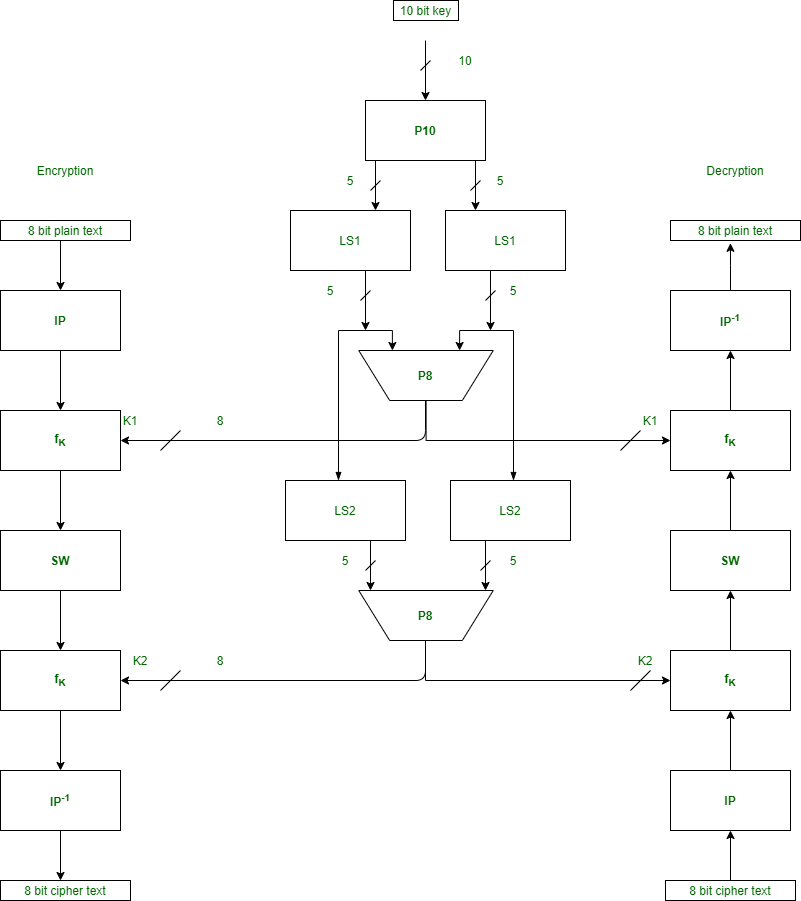Components :
S-DES encryption involves four functions –

1. Initial permutation(IP) –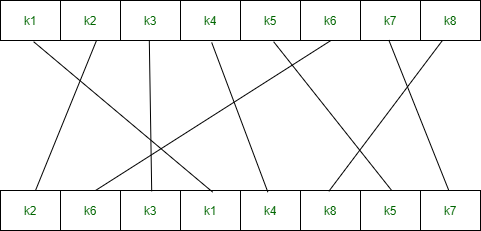2. Complex function (fk) –
It is the combination of permutation and substitution functions. The below image represents a round of encryption and decryption. This round is repeated twice in each encryption and decryption.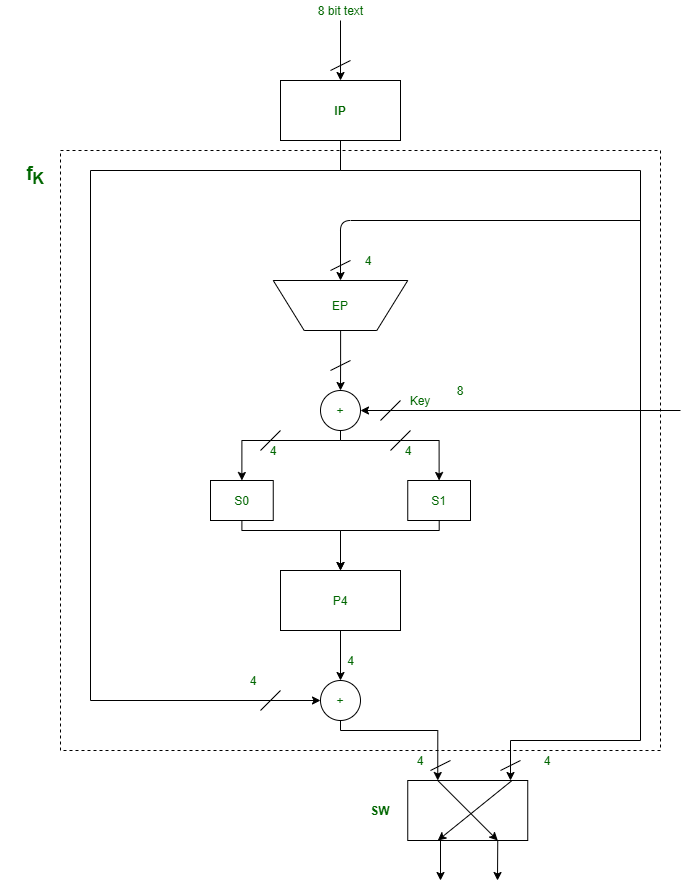Components in fk are –
a. Expanded Permutation (EP) –
It takes a 4-bit input and converts it into an 8-bit output.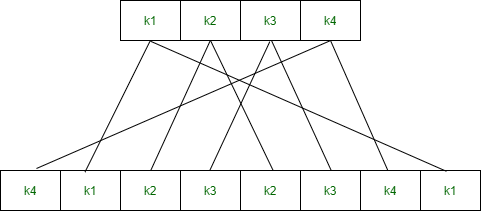b. S-boxes (S0 and S1) –
It is a basic component of a symmetric key algorithm that performs substitution.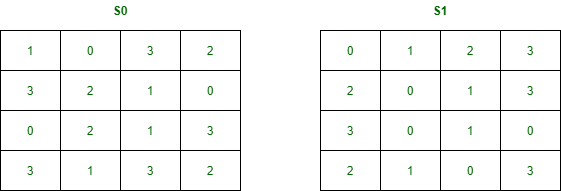c. Permutation P4 –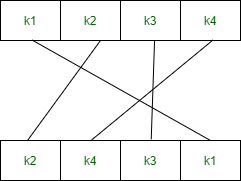3. Switch (SW) –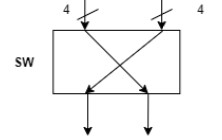4. Inverse of Initial Permutation (IP-1) –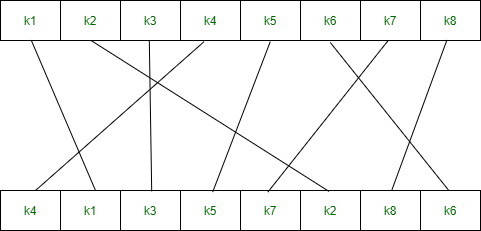First, we need to generate 2 keys before encryption.

`Consider, the entered 10-bit key is - 1 0 1 0 0 0 0 0 1 0`

Therefore,

```Key-1 is - 1 0 1 0 0 1 0 0
Key-2 is - 0 1 0 0 0 0 1 1```

#### Encryption –

`Entered 8-bit plaintext is - 1 0 0 1 0 1 1 1`

Step-1:
We perform initial permutation on our 8-bit plain text using the IP table. The initial permutation is defined as –

```IP(k1, k2, k3, k4, k5, k6, k7, k8) = (k2, k6, k3, k1, k4, k8, k5, k7)
After ip = 0 1 0 1 1 1 0 1```

Step-2:
After the initial permutation, we get an 8-bit block of text which we divide into 2 halves of 4 bit each.

`l = 0 1 0 1  and r = 1 1 0 1`

On the right half, we perform expanded permutation using EP table which converts 4 bits into 8 bits. Expand permutation is defined as –

`EP(k1, k2, k3, k4) = (k4, k1, k2, k3, k2, k3, k4, k1)`
`After ep = 1 1 1 0 1 0 1 1`

We perform XOR operation using the first key K1 with the output of expanded permutation.

```Key-1 is - 1 0 1 0 0 1 0 0
(1 0 1 0 0 1 0 0) XOR (1 1 1 0 1 0 1 1) =  0 1 0 0 1 1 1 1
After XOR operation with 1st Key = 0 1 0 0 1 1 1 1```

Again we divide the output of XOR into 2 halves of 4 bit each.

`l = 0 1 0 0  and r = 1 1 1 1`

We take the first and fourth bit as row and the second and third bit as a column for our S boxes.

```S0 = [1,0,3,2
3,2,1,0
0,2,1,3
3,1,3,2]

S1=  [0,1,2,3
2,0,1,3
3,0,1,0
2,1,0,3]

For l = 0 1 0 0
row = 00 = 0, column = 10 = 2
S0 = 3 = 11

For r = 1 1 1 1
row = 11 = 3, column = 11 = 3
S1 = 3 = 11

After first S-Boxes combining S0 and S1 = 1 1 1 1```

S boxes gives a 2-bit output which we combine to get 4 bits and then perform permutation using the P4 table. P4 is defined as –

```P4(k1, k2, k3, k4) = (k2, k4, k3, k1)
After P4 = 1 1 1 1```

We XOR the output of the P4 table with the left half of the initial permutation table i.e. IP table.

```(0 1 0 1) XOR (1 1 1 1) = 1 0 1 0
After XOR operation with left nibble of after ip = 1 0 1 0```

We combine both halves i.e. right half of initial permutation and output of ip.

```Combine 1 1 0 1 and 1 0 1 0
After combine = 1 0 1 0 1 1 0 1```

Step-3:
Now, divide the output into two halves of 4 bit each. Combine them again, but now the left part should become right and the right part should become left.

`After step 3 = 1 1 0 1 1 0 1 0`

Step-4:
Again perform step 2, but this time while doing XOR operation after expanded permutation use key 2 instead of key 1.

```Expand permutation is defined as - 4 1 2 3 2 3 4 1
After second ep = 0 1 0 1 0 1 0 1
After XOR operation with 2nd Key = 0 0 0 1 0 1 1 0
After second S-Boxes = 1 1 1 1

P4 is defined as - 2 4 3 1
After P4 = 1 1 1 1

After XOR operation with left nibble of after first part = 0 0 1 0
After second part = 0 0 1 0 1 0 1 0

l = 1 1 0 1  and r = 1 0 1 0```

On the right half, we perform expanded permutation using EP table which converts 4 bits into 8 bits. Expand permutation is defined as –

```EP(k1, k2, k3, k4) = (k4, k1, k2, k3, k2, k3, k4, k1)
After second ep = 0 1 0 1 0 1 0 1```

We perform XOR operation using second key K2 with the output of expanded permutation.

```Key-2 is - 0 1 0 0 0 0 1 1
(0 1 0 0 0 0 1 1) XOR (0 1 0 1 0 1 0 1) =  0 0 0 1 0 1 1 0
After XOR operation with 2nd Key = 0 0 0 1 0 1 1 0```

Again we divide the output of XOR into 2 halves of 4 bit each.

`l = 0 0 0 1  and r = 0 1 1 0`

We take the first and fourth bit as row and the second and third bit as a column for our S boxes.

```S0 = [1,0,3,2
3,2,1,0
0,2,1,3
3,1,3,2]

S1 = [0,1,2,3
2,0,1,3
3,0,1,0
2,1,0,3]

For l = 0 0 0 1
row = 01 = 1 , column = 00 = 0
S0 = 3 = 11

For r = 0 1 1 0
row = 00 = 0 , column = 11 = 3
S1 = 3 = 11

After first S-Boxes combining S0 and S1 = 1 1 1 1```

S boxes gives a 2-bit output which we combine to get 4 bits and then perform permutation using the P4 table. P4 is defined as –

```P4(k1, k2, k3, k4) = (k2, k4, k3, k1)
After P4 = 1 1 1 1```

We XOR the output of the P4 table with the left half of the initial permutation table i.e. IP table.

```(1 1 0 1) XOR (1 1 1 1) = 0 0 1 0
After XOR operation with left nibble of after first part = 0 0 1 0```

We combine both halves i.e. right half of initial permutation and output of ip.

```Combine 1 0 1 0 and 0 0 1 0
After combine = 0 0 1 0 1 0 1 0
After second part = 0 0 1 0 1 0 1 0```

Step-5:
Perform inverse initial permutation. The output of this table is the cipher text of 8 bit.

`Output of step 4 : 0 0 1 0 1 0 1 0`

Inverse Initial permutation is defined as –

`IP-1(k1, k2, k3, k4, k5, k6, k7, k8) = (k4, k1, k3, k5, k7, k2, k8, k6)`

8-bit Cipher Text will be = 0 0 1 1 1 0 0 0

## Java

 `/*package whatever //do not write package name here */` `import` `java.io.*;` `public` `class` `GFG {``    ``// int key[]= {0,0,1,0,0,1,0,1,1,1};``    ``int` `key[] = {``        ``1``, ``0``, ``1``, ``0``, ``0``, ``0``, ``0``, ``0``, ``1``, ``0``    ``}; ``// extra example for checking perpose``    ``int` `P10[] = { ``3``, ``5``, ``2``, ``7``, ``4``, ``10``, ``1``, ``9``, ``8``, ``6` `};``    ``int` `P8[] = { ``6``, ``3``, ``7``, ``4``, ``8``, ``5``, ``10``, ``9` `};` `    ``int` `key1[] = ``new` `int``[``8``];``    ``int` `key2[] = ``new` `int``[``8``];` `    ``int``[] IP = { ``2``, ``6``, ``3``, ``1``, ``4``, ``8``, ``5``, ``7` `};``    ``int``[] EP = { ``4``, ``1``, ``2``, ``3``, ``2``, ``3``, ``4``, ``1` `};``    ``int``[] P4 = { ``2``, ``4``, ``3``, ``1` `};``    ``int``[] IP_inv = { ``4``, ``1``, ``3``, ``5``, ``7``, ``2``, ``8``, ``6` `};` `    ``int``[][] S0 = { { ``1``, ``0``, ``3``, ``2` `},``                   ``{ ``3``, ``2``, ``1``, ``0` `},``                   ``{ ``0``, ``2``, ``1``, ``3` `},``                   ``{ ``3``, ``1``, ``3``, ``2` `} };``    ``int``[][] S1 = { { ``0``, ``1``, ``2``, ``3` `},``                   ``{ ``2``, ``0``, ``1``, ``3` `},``                   ``{ ``3``, ``0``, ``1``, ``0` `},``                   ``{ ``2``, ``1``, ``0``, ``3` `} };` `    ``//    this function basically generates the key(key1 and``    ``//key2)     using P10 and P8 with (1 and 2)left shifts` `    ``void` `key_generation()``    ``{``        ``int` `key_[] = ``new` `int``[``10``];` `        ``for` `(``int` `i = ``0``; i < ``10``; i++) {``            ``key_[i] = key[P10[i] - ``1``];``        ``}` `        ``int` `Ls[] = ``new` `int``[``5``];``        ``int` `Rs[] = ``new` `int``[``5``];` `        ``for` `(``int` `i = ``0``; i < ``5``; i++) {``            ``Ls[i] = key_[i];``            ``Rs[i] = key_[i + ``5``];``        ``}` `        ``int``[] Ls_1 = shift(Ls, ``1``);``        ``int``[] Rs_1 = shift(Rs, ``1``);` `        ``for` `(``int` `i = ``0``; i < ``5``; i++) {``            ``key_[i] = Ls_1[i];``            ``key_[i + ``5``] = Rs_1[i];``        ``}` `        ``for` `(``int` `i = ``0``; i < ``8``; i++) {``            ``key1[i] = key_[P8[i] - ``1``];``        ``}` `        ``int``[] Ls_2 = shift(Ls, ``2``);``        ``int``[] Rs_2 = shift(Rs, ``2``);` `        ``for` `(``int` `i = ``0``; i < ``5``; i++) {``            ``key_[i] = Ls_2[i];``            ``key_[i + ``5``] = Rs_2[i];``        ``}` `        ``for` `(``int` `i = ``0``; i < ``8``; i++) {``            ``key2[i] = key_[P8[i] - ``1``];``        ``}` `        ``System.out.println(``"Your Key-1 :"``);` `        ``for` `(``int` `i = ``0``; i < ``8``; i++)``            ``System.out.print(key1[i] + ``" "``);` `        ``System.out.println();``        ``System.out.println(``"Your Key-2 :"``);` `        ``for` `(``int` `i = ``0``; i < ``8``; i++)``            ``System.out.print(key2[i] + ``" "``);``    ``}` `    ``//    this function is use full for shifting(circular) the``    ``//array n position towards left` `    ``int``[] shift(``int``[] ar, ``int` `n)``    ``{``        ``while` `(n > ``0``) {``            ``int` `temp = ar[``0``];``            ``for` `(``int` `i = ``0``; i < ar.length - ``1``; i++) {``                ``ar[i] = ar[i + ``1``];``            ``}``            ``ar[ar.length - ``1``] = temp;``            ``n--;``        ``}``        ``return` `ar;``    ``}` `    ``//    this is main encryption function takes plain text as``    ``//input     uses another functions and returns the array of``    ``//cipher text` `    ``int``[] encryption(``int``[] plaintext)``    ``{``        ``int``[] arr = ``new` `int``[``8``];` `        ``for` `(``int` `i = ``0``; i < ``8``; i++) {``            ``arr[i] = plaintext[IP[i] - ``1``];``        ``}``        ``int``[] arr1 = function_(arr, key1);` `        ``int``[] after_swap = swap(arr1, arr1.length / ``2``);` `        ``int``[] arr2 = function_(after_swap, key2);` `        ``int``[] ciphertext = ``new` `int``[``8``];` `        ``for` `(``int` `i = ``0``; i < ``8``; i++) {``            ``ciphertext[i] = arr2[IP_inv[i] - ``1``];``        ``}` `        ``return` `ciphertext;``    ``}` `    ``// decimal to binary string 0-3` `    ``String binary_(``int` `val)``    ``{``        ``if` `(val == ``0``)``            ``return` `"00"``;``        ``else` `if` `(val == ``1``)``            ``return` `"01"``;``        ``else` `if` `(val == ``2``)``            ``return` `"10"``;``        ``else``            ``return` `"11"``;``    ``}` `    ``//    this function is doing core things like expansion``    ``//    then xor with desired key then S0 and S1``    ``//substitution     P4 permutation and again xor     we have used``    ``//this function 2 times(key-1 and key-2) during``    ``//encryption and     2 times(key-2 and key-1) during``    ``//decryption` `    ``int``[] function_(``int``[] ar, ``int``[] key_)``    ``{` `        ``int``[] l = ``new` `int``[``4``];``        ``int``[] r = ``new` `int``[``4``];` `        ``for` `(``int` `i = ``0``; i < ``4``; i++) {``            ``l[i] = ar[i];``            ``r[i] = ar[i + ``4``];``        ``}` `        ``int``[] ep = ``new` `int``[``8``];` `        ``for` `(``int` `i = ``0``; i < ``8``; i++) {``            ``ep[i] = r[EP[i] - ``1``];``        ``}` `        ``for` `(``int` `i = ``0``; i < ``8``; i++) {``            ``ar[i] = key_[i] ^ ep[i];``        ``}` `        ``int``[] l_1 = ``new` `int``[``4``];``        ``int``[] r_1 = ``new` `int``[``4``];` `        ``for` `(``int` `i = ``0``; i < ``4``; i++) {``            ``l_1[i] = ar[i];``            ``r_1[i] = ar[i + ``4``];``        ``}` `        ``int` `row, col, val;` `        ``row = Integer.parseInt(``""` `+ l_1[``0``] + l_1[``3``], ``2``);``        ``col = Integer.parseInt(``""` `+ l_1[``1``] + l_1[``2``], ``2``);``        ``val = S0[row][col];``        ``String str_l = binary_(val);` `        ``row = Integer.parseInt(``""` `+ r_1[``0``] + r_1[``3``], ``2``);``        ``col = Integer.parseInt(``""` `+ r_1[``1``] + r_1[``2``], ``2``);``        ``val = S1[row][col];``        ``String str_r = binary_(val);` `        ``int``[] r_ = ``new` `int``[``4``];``        ``for` `(``int` `i = ``0``; i < ``2``; i++) {``            ``char` `c1 = str_l.charAt(i);``            ``char` `c2 = str_r.charAt(i);``            ``r_[i] = Character.getNumericValue(c1);``            ``r_[i + ``2``] = Character.getNumericValue(c2);``        ``}``        ``int``[] r_p4 = ``new` `int``[``4``];``        ``for` `(``int` `i = ``0``; i < ``4``; i++) {``            ``r_p4[i] = r_[P4[i] - ``1``];``        ``}` `        ``for` `(``int` `i = ``0``; i < ``4``; i++) {``            ``l[i] = l[i] ^ r_p4[i];``        ``}` `        ``int``[] ouptput = ``new` `int``[``8``];``        ``for` `(``int` `i = ``0``; i < ``4``; i++) {``            ``ouptput[i] = l[i];``            ``ouptput[i + ``4``] = r[i];``        ``}``        ``return` `ouptput;``    ``}` `    ``//    this function swaps the nibble of size n(4)` `    ``int``[] swap(``int``[] aray, ``int` `n)``    ``{``        ``int``[] l = ``new` `int``[n];``        ``int``[] r = ``new` `int``[n];` `        ``for` `(``int` `i = ``0``; i < n; i++) {``            ``l[i] = aray[i];``            ``r[i] = aray[i + n];``        ``}` `        ``int``[] output = ``new` `int``[``2` `* n];``        ``for` `(``int` `i = ``0``; i < n; i++) {``            ``output[i] = r[i];``            ``output[i + n] = l[i];``        ``}` `        ``return` `output;``    ``}` `    ``//    this is main decryption function``    ``//    here we have used all previously defined function``    ``//    it takes cipher text as input and returns the array``    ``//of     decrypted text` `    ``int``[] decryption(``int``[] ar)``    ``{``        ``int``[] arr = ``new` `int``[``8``];` `        ``for` `(``int` `i = ``0``; i < ``8``; i++) {``            ``arr[i] = ar[IP[i] - ``1``];``        ``}` `        ``int``[] arr1 = function_(arr, key2);` `        ``int``[] after_swap = swap(arr1, arr1.length / ``2``);` `        ``int``[] arr2 = function_(after_swap, key1);` `        ``int``[] decrypted = ``new` `int``[``8``];` `        ``for` `(``int` `i = ``0``; i < ``8``; i++) {``            ``decrypted[i] = arr2[IP_inv[i] - ``1``];``        ``}` `        ``return` `decrypted;``    ``}` `    ``public` `static` `void` `main(String[] args)``    ``{` `        ``GFG obj = ``new` `GFG();` `        ``obj.key_generation(); ``// call to key generation``                              ``// function` `        ``// int []plaintext= {1,0,1,0,0,1,0,1};``        ``int``[] plaintext = {``            ``1``, ``0``, ``0``, ``1``, ``0``, ``1``, ``1``, ``1``        ``}; ``// extra example for checking perpose` `        ``System.out.println();``        ``System.out.println(``"Your plain Text is :"``);``        ``for` `(``int` `i = ``0``; i < ``8``; i++) ``// printing the``                                    ``// plaintext``            ``System.out.print(plaintext[i] + ``" "``);` `        ``int``[] ciphertext = obj.encryption(plaintext);` `        ``System.out.println();``        ``System.out.println(``            ``"Your cipher Text is :"``); ``// printing the cipher``                                      ``// text``        ``for` `(``int` `i = ``0``; i < ``8``; i++)``            ``System.out.print(ciphertext[i] + ``" "``);` `        ``int``[] decrypted = obj.decryption(ciphertext);` `        ``System.out.println();``        ``System.out.println(``            ``"Your decrypted Text is :"``); ``// printing the``                                         ``// decrypted text``        ``for` `(``int` `i = ``0``; i < ``8``; i++)``            ``System.out.print(decrypted[i] + ``" "``);``    ``}``}` `//Omkar Varhadi`
Output
```Your Key-1 :
1 0 1 0 0 1 0 0
Your Key-2 :
0 1 0 0 0 0 1 1
Your plain Text is :
1 0 0 1 0 1 1 1
Your cipher Text is :
0 0 1 1 1 0 0 0
Your decrypted Text is :
1 0 0 1 0 1 1 1 ```

Attention reader! Don’t stop learning now. Get hold of all the important CS Theory concepts for SDE interviews with the CS Theory Course at a student-friendly price and become industry ready.

My Personal Notes arrow_drop_up